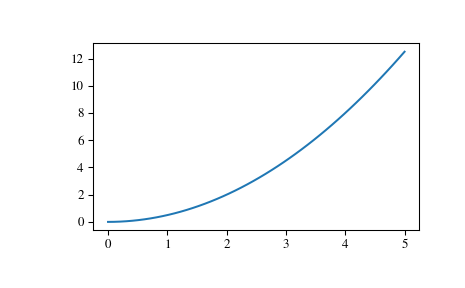# scipy.signal.lsim¶

scipy.signal.lsim(system, U, T, X0=None, interp=True)[source]

Simulate output of a continuous-time linear system.

Parameters: system : an instance of the LTI class or a tuple describing the system. The following gives the number of elements in the tuple and the interpretation: 1: (instance of lti) 2: (num, den) 3: (zeros, poles, gain) 4: (A, B, C, D) U : array_like An input array describing the input at each time T (interpolation is assumed between given times). If there are multiple inputs, then each column of the rank-2 array represents an input. If U = 0 or None, a zero input is used. T : array_like The time steps at which the input is defined and at which the output is desired. Must be nonnegative, increasing, and equally spaced. X0 : array_like, optional The initial conditions on the state vector (zero by default). interp : bool, optional Whether to use linear (True, the default) or zero-order-hold (False) interpolation for the input array. T : 1D ndarray Time values for the output. yout : 1D ndarray System response. xout : ndarray Time evolution of the state vector.

Notes

If (num, den) is passed in for system, coefficients for both the numerator and denominator should be specified in descending exponent order (e.g. s^2 + 3s + 5 would be represented as [1, 3, 5]).

Examples

Simulate a double integrator y’’ = u, with a constant input u = 1

>>> from scipy import signal
>>> system = signal.lti([[0., 1.], [0., 0.]], [[0.], [1.]], [[1., 0.]], 0.)
>>> t = np.linspace(0, 5)
>>> u = np.ones_like(t)
>>> tout, y, x = signal.lsim(system, u, t)
>>> import matplotlib.pyplot as plt
>>> plt.plot(t, y)#### Previous topic

scipy.signal.ZerosPolesGain.to_zpk

#### Next topic

scipy.signal.lsim2